# TypeError: 'list' object cannot be interpreted as an integerLast updated: Feb 1, 2023
8 min## #TypeError: 'list' object cannot be interpreted as an integer

The Python "TypeError: 'list' object cannot be interpreted as an integer" occurs when we pass a list to a function that expects an integer argument, e.g. `range()`.

To solve the error, either pass the length of the list or pass an integer to the function.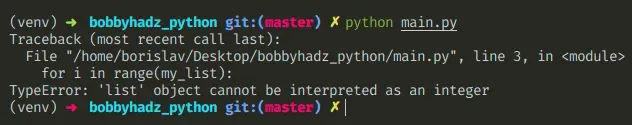Here is an example of how the error occurs.

main.py
```Copied!```my_list = ['a', 'b', 'c']

# 👇️ expects to get called with an integer but is called with a list

# ⛔️ TypeError: 'list' object cannot be interpreted as an integer
for i in range(my_list):
print(i)
``````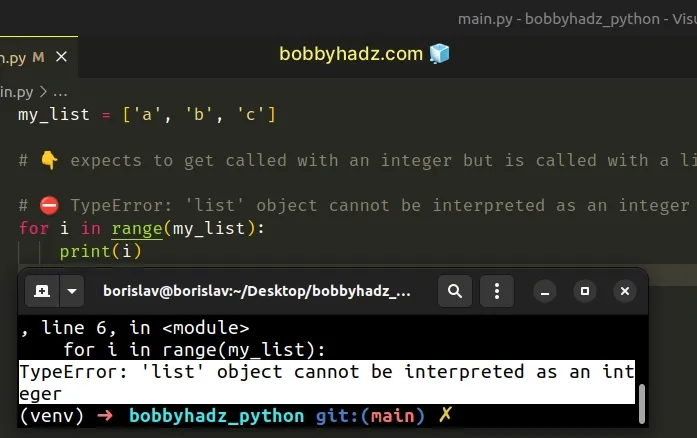The `range` function expects an integer as an argument, however, we called the function with a list.

## #Pass an integer instead of a list to the function

To solve the error, we have to pass an integer instead of a list to the function.

For example, you can pass the length of the list as an argument to the function.

main.py
```Copied!```my_list = ['a', 'b', 'c']

for i in range(len(my_list)):
print(i)  # 👉️ 0, 1, 2

print(len(my_list)) # 👉️ 3
``````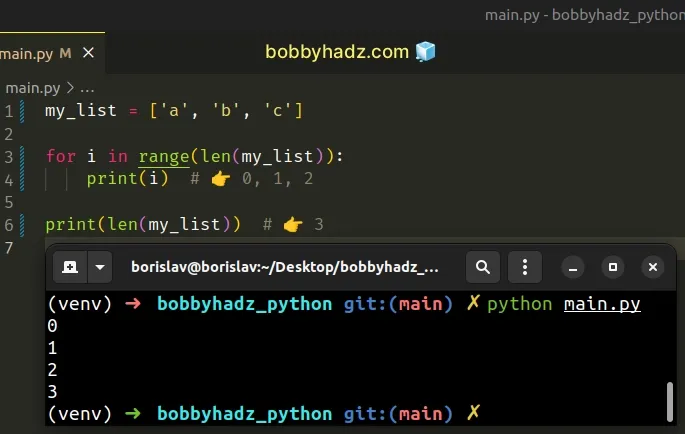The len() function returns the length (the number of items) of an object.

We passed the length of the list (3) to the `range()` function to create a range object that starts at `0` and goes to `2`.

## #Iterating over a list in Python

If you meant to iterate over the list, remove the call to `range()`.

main.py
```Copied!```my_list = ['a', 'b', 'c']

# ✅ iterating over a list

for item in my_list:
print(item)  # 👉️ 'a', 'b', 'c'
``````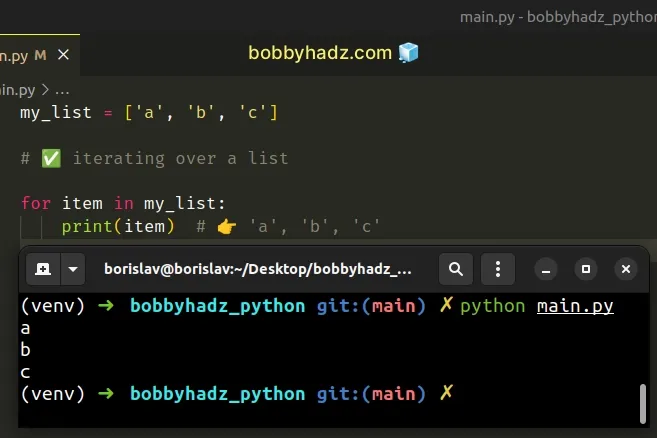If you need access to the index of the current iteration when iterating over a list, use the `enumerate()` function.

main.py
```Copied!```my_list = ['a', 'b', 'c']

for idx, item in enumerate(my_list):
print(idx, item)  # 👉️ 0 a, 1 b, 2 c
``````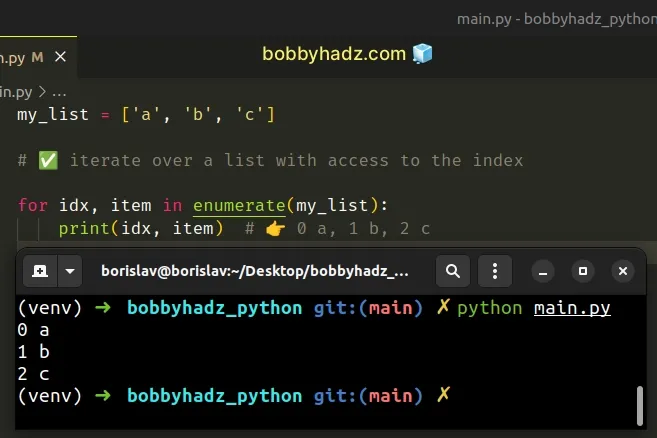The enumerate function takes an iterable and returns an enumerate object containing tuples where the first element is the index and the second is the corresponding item.

We got the error because the `range()` function takes integers as arguments, but we passed a list to the function.

The range function is commonly used for looping a specific number of times in `for` loops and takes the following parameters:

NameDescription
`start`An integer representing the start of the range (defaults to `0`)
`stop`Go up to, but not including the provided integer
`step`Range will consist of every N numbers from `start` to `stop` (defaults to `1`)
main.py
```Copied!```print(list(range(3))) # 👉️ [0, 1, 2]

print(list(range(1, 4))) # 👉️ [1, 2, 3]

print(list(range(1, 4))) # 👉️ [1, 2, 3]
``````

If you only pass a single argument to the `range()` constructor, it is considered to be the value for the `stop` parameter.

## #Calling the `list.pop()` function with a list instead of an integer

Make sure you aren't calling the `list.pop()` method with a list instead of an integer.

main.py
```Copied!```a_list = ['a', 'b', 'c']

# ⛔️ TypeError: 'list' object cannot be interpreted as an integer
a_list.pop()
``````

The `list.pop` method removes the item at the given position in the list and returns it.

You should pass an integer in the call to the `pop()` method to solve the error.

main.py
```Copied!```a_list = ['a', 'b', 'c']

a_list.pop(1)

print(a_list) # 👉️ ['a', 'c']
``````

If no index is specified, the `pop()` method removes and returns the last item in the list.

main.py
```Copied!```my_list = ['bobby', 'hadz', 'com']

my_list.pop()

``````
If you are getting the error with a different function, you either have to pass the length of the list `len(my_list)` as an argument to the function or figure out where the variable gets assigned a list in your code.

## #Reassigning a variable to an integer by mistake

Make sure you aren't declaring a variable that stores an integer initially and overriding it somewhere in your code.

main.py
```Copied!```my_int = 10

# 👇️ reassigned variable to a list by mistake
my_int = ['a', 'b', 'c']

# 👇️ function expects to be called with an integer argument
# ⛔️ TypeError: 'list' object cannot be interpreted as an integer
result = range(my_int)
``````

We initially set the `my_int` variable to an integer but later reassigned it to a list.

You have to track down where the variable got assigned a list and correct the assignment.

## #Checking what type a variable stores

You can use the `type` and `isinstance` functions if you aren't sure what type a variable stores.

main.py
```Copied!```a_list = ['a', 'b', 'c']

print(type(a_list))  # 👉️  <class 'list'>
print(isinstance(a_list, list))  # 👉️ True

an_int = 100

print(type(an_int))  # 👉️ <class 'int'>
print(isinstance(an_int, int))  # 👉️ True
``````

The type class returns the type of an object.

The isinstance function returns `True` if the passed-in object is an instance or a subclass of the passed-in class.

## #TypeError: tuple object cannot be interpreted as an integer

The Python "TypeError: 'tuple' object cannot be interpreted as an integer" occurs when we pass a tuple to a function that expects an integer argument.

To solve the error, either unpack the tuple or access the tuple element at a specific index.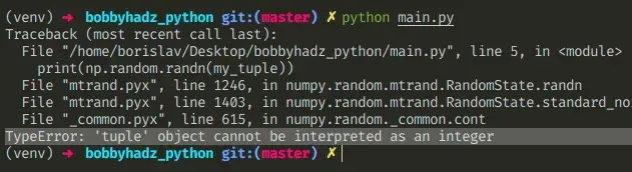Here is an example of how the error occurs.

main.py
```Copied!```import numpy as np

my_tuple = (2, 4)

# ⛔️ TypeError: 'tuple' object cannot be interpreted as an integer
print(np.random.randn(my_tuple))
``````

And here is another example.

main.py
```Copied!```my_tuple = (2, 4)

# ⛔️ TypeError: 'tuple' object cannot be interpreted as an integer
for i in range(my_tuple):
print(i)
``````

We have a tuple that contains 2 elements but the `random.randn()` and `range()` functions expect integer arguments instead of a tuple.

If you wrapped the integer arguments in two sets of curly braces, remove one set as this creates a tuple.
main.py
```Copied!```my_tuple = (1, 2)

print(my_tuple) # 👉️ (1, 2)
print(type(my_tuple)) # 👉️ <class 'tuple'>
``````

## #Access the tuple at a specific index

One way to solve the error is to access the tuple at a specific index if your tuple stores integers.

main.py
```Copied!```import numpy as np

my_tuple = (2, 4)

# ✅ works
print(np.random.randn(my_tuple, my_tuple))
``````

And here is an example that uses the built-in `range()` function.

main.py
```Copied!```my_tuple = (2, 4)

# ✅ works
for i in range(my_tuple, my_tuple):
print(i)
``````
Our tuple stores integer values, so we can access it at a specific index and pass the integers as arguments to the function.

## #Using unpacking in the call to the function

If your tuple stores exactly as many elements as the function's arguments, you can unpack the tuple in the call to the function.

main.py
```Copied!```import numpy as np

my_tuple = (2, 4)

# ✅ works
print(np.random.randn(*my_tuple))

# ✅ works
for i in range(*my_tuple):
print(i)
``````

You can imagine that the `*my_tuple` syntax unpacks the two elements of the tuple and passes them as individual arguments to the function.

We end up passing 2 integers as arguments to the function, instead of a single tuple.

## #Setting an integer variable to a tuple by mistake

Make sure you aren't declaring a variable that stores an integer initially and overriding it somewhere in your code.

main.py
```Copied!```my_int = 10

my_int = 15, # 👈️ note: the trailing comma creates a tuple

print(type(my_int))  # 👉️ <class 'tuple'>

# ⛔️ TypeError: 'tuple' object cannot be interpreted as an integer
for i in range(my_int):
print(i)
``````

We initially set the `my_int` variable to an integer but later reassigned it to a tuple.

In this case, you have to track down where the variable got assigned a tuple and correct the assignment.

## #How tuples are constructed in Python

Tuples are constructed in multiple ways:

• Using a pair of parentheses `()` creates an empty tuple
• Using a trailing comma - `a,` or `(a,)`
• Separating items with commas - `a, b` or `(a, b)`
• Using the `tuple()` constructor

## #NoneType object cannot be interpreted as an integer - Python

The Python "TypeError: 'NoneType' object cannot be interpreted as an integer" occurs when we pass a `None` value to a function that expects an integer argument.

To solve the error, track down where the `None` value comes from and pass an integer to the function.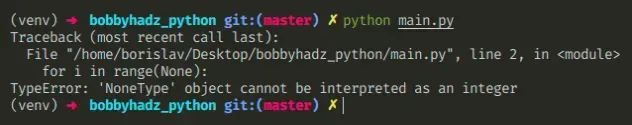Here is an example of how the error occurs.

main.py
```Copied!```# ⛔️ TypeError: 'tuple' object cannot be interpreted as an integer
for i in range(None):
print(i)
``````

We passed a `None` value to the `range()` constructor which expects to get called with an integer.

## #Checking if the variable is not None before calling the function

You can check if the variable doesn't store a None value before calling the function.

main.py
```Copied!```my_num = None

if my_num is not None:
for i in range(my_num):
print(i)
else:
print('variable is None')
``````

The `if` block will run only if the `my_num` variable doesn't store a `None` value, otherwise, the `else` block runs.

You can also provide a default value of the variable is `None`.

main.py
```Copied!```my_num = None

if my_num is None:
my_num = 3

for i in range(my_num):
print(i) # 👉️ 0, 1, 2
``````

We check if the variable is `None` and if it is, we set the variable to `3`.

## #Track down where the variable got assigned a `None` value

To solve the error, we have to figure out where the `None` value comes from and correct the assignment.

The most common source of a `None` value (other than an explicit assignment) is a function that doesn't return anything.

main.py
```Copied!```# 👇️ this function returns None
def get_num(a, b):
print(a + b)

# ⛔️ TypeError: 'NoneType' object cannot be interpreted as an integer
for i in range(get_num(5, 5)):
print(i)
``````

Notice that our `get_num` function doesn't explicitly return a value, so it implicitly returns None.

Make sure to return a value from the function.

main.py
```Copied!```def get_num(a, b):
return a + b

for i in range(get_num(1, 2)):
print(i)  # 👉️ 0, 1, 2
``````

We used a return statement to return a value from the function and passed an integer to the `range()` function.

The error occurs for multiple reasons:

1. Having a function that doesn't return anything (returns `None` implicitly).
2. Explicitly setting a variable to `None`.
3. Assigning a variable to the result of calling a built-in function that doesn't return anything.
4. Having a function that only returns a value if a certain condition is met.
Avoid assignment with methods that mutate the original object in place, as most of them don't return a value and implicitly return `None`.

## #A function returning an integer only if a condition is met

Another common cause of the error is having a function that returns a value only if a condition is met.

main.py
```Copied!```def get_num(a):
if a > 100:
return a

result = get_num(5)

print(result)  # 👉️ None
``````

The `if` statement in the `get_num` function is only run if the passed in argument is greater than `100`.

In all other cases, the function doesn't return anything and ends up implicitly returning `None`.

To solve the error in this scenario, you either have to check if the function didn't return `None` or return a default value if the condition is not met.

main.py
```Copied!```def get_num(a):
if a > 100:
return a
return 0 # 👈️ returns 0 if condition not met

result = get_num(5)

print(result)  # 👉️ 0
``````

Now the function is guaranteed to return a value regardless if the condition is met.

I wrote a book in which I share everything I know about how to become a better, more efficient programmer.You can use the search field on my Home Page to filter through all of my articles.